Ex 13.1

Chapter 13 Class 12 Probability
Serial order wise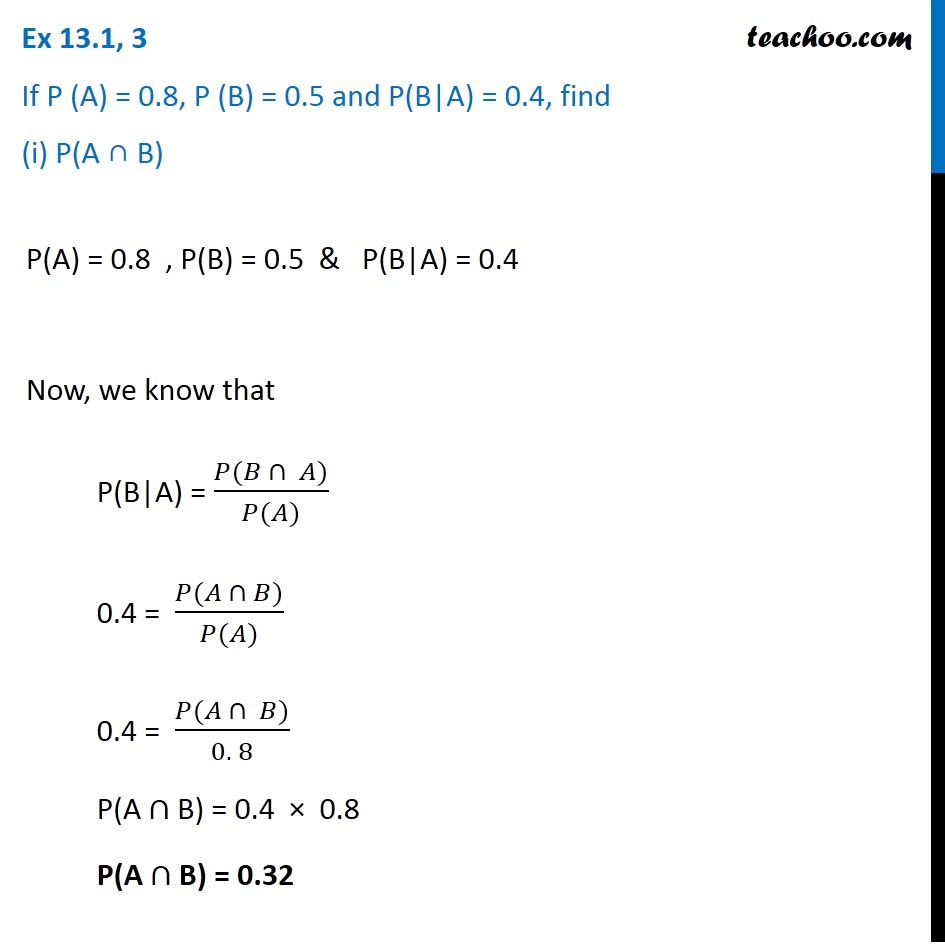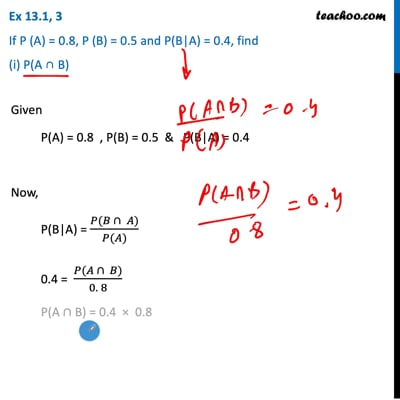This video is only available for Teachoo black users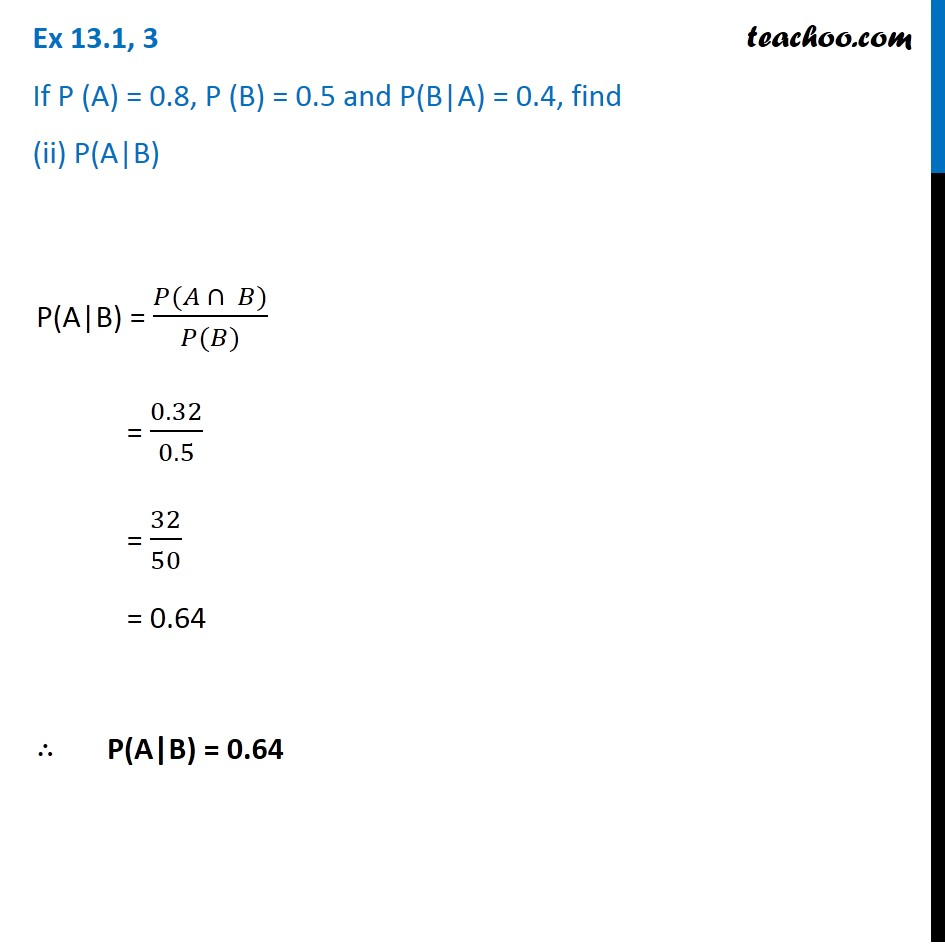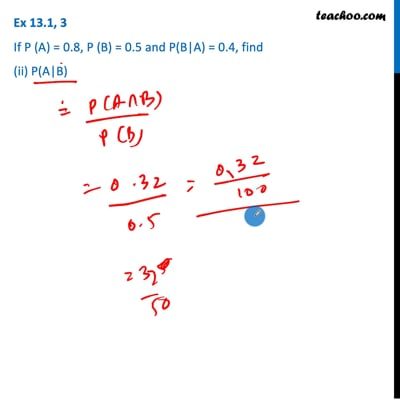This video is only available for Teachoo black users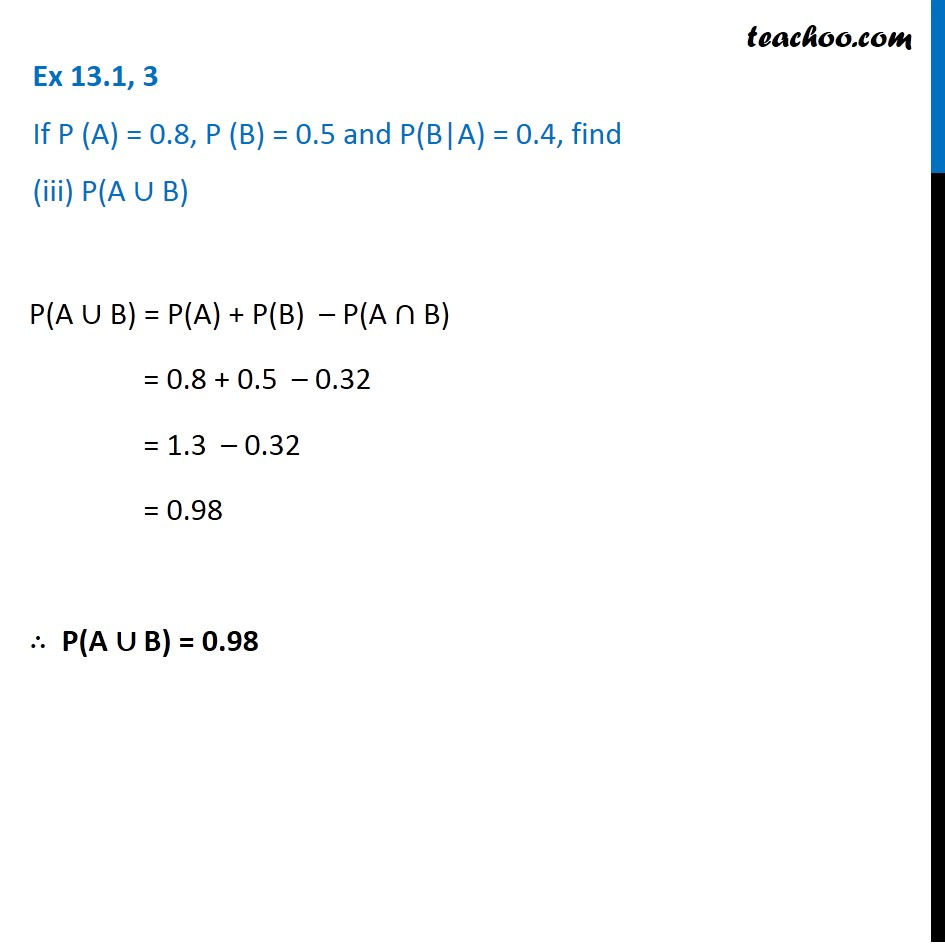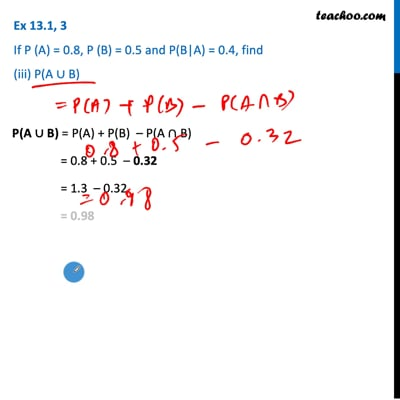This video is only available for Teachoo black users

Get live Maths 1-on-1 Classs - Class 6 to 12

### Transcript

Ex 13.1, 3 If P (A) = 0.8, P (B) = 0.5 and P(B|A) = 0.4, find (i) P(A ∩ B)P(A) = 0.8 , P(B) = 0.5 & P(B|A) = 0.4 Now, we know that P(B|A) = (𝑃(𝐵 ∩ 𝐴))/(𝑃(𝐴)) 0.4 = (𝑃(𝐴 ∩ 𝐵))/(𝑃(𝐴)) 0.4 = (𝑃(𝐴 ∩ 𝐵))/(0. 8) P(A ∩ B) = 0.4 × 0.8 P(A ∩ B) = 0.32 Ex 13.1, 3 If P (A) = 0.8, P (B) = 0.5 and P(B|A) = 0.4, find (ii) P(A|B)P(A|B) = (𝑃(𝐴 ∩ 𝐵))/(𝑃(𝐵)) = 0.32/0.5 = 32/50 = 0.64 ∴ P(A|B) = 0.64 Ex 13.1, 3 If P (A) = 0.8, P (B) = 0.5 and P(B|A) = 0.4, find (iii) P(A ∪ B)P(A ∪ B) = P(A) + P(B) – P(A ∩ B) = 0.8 + 0.5 – 0.32 = 1.3 – 0.32 = 0.98 ∴ P(A ∪ B) = 0.98